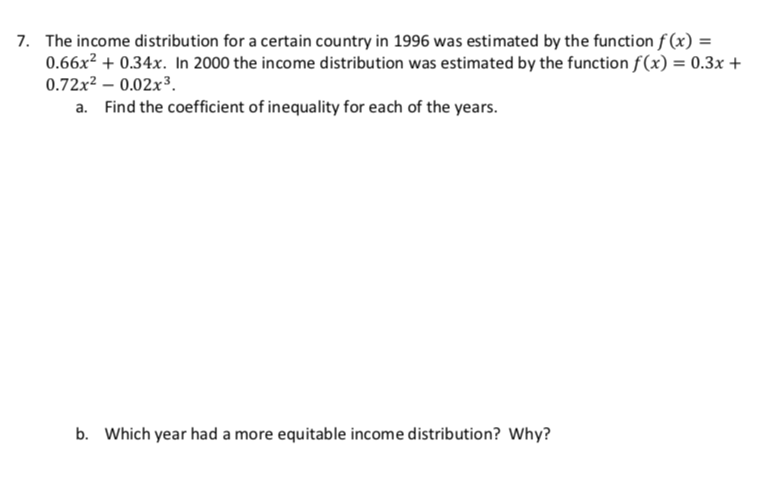# The income distribution for a certain country in 1996 was estimated by the function f(x) =0.66x2 +0.34x. In 2000 the income distribution was estimated by the function f(x) = 0.3x0.72x2 -0.02x3a. Find the coefficient of inequality for each of the years7.b.Which year had a more equitable income distribution? Why?

Question
39 viewshelp_outlineImage TranscriptioncloseThe income distribution for a certain country in 1996 was estimated by the function f(x) = 0.66x2 +0.34x. In 2000 the income distribution was estimated by the function f(x) = 0.3x 0.72x2 -0.02x3 a. Find the coefficient of inequality for each of the years 7. b. Which year had a more equitable income distribution? Why? fullscreen
check_circle

Step 1

Income distribution defines the two-parameter model for the Lorenz Curve which shows the cumulative percentage of national income held by the bottom % of the population. The L(x) curve gives the graphical representation of the distribution of income and thus depicts income in equality present in an economy.

Step 2

The equation (1) gives income distribution function of estimated L(X) for the year 1996.

And equation (2) gives the income distribution in 2000.

Step 3

To calculate Gini coefficient that gives the inequ...

### Want to see the full answer?

See Solution

#### Want to see this answer and more?

Solutions are written by subject experts who are available 24/7. Questions are typically answered within 1 hour.*

See Solution
*Response times may vary by subject and question.
Tagged in